# Graph colouring

(diff) ← Older revision | Latest revision (diff) | Newer revision → (diff)

An assignment of colours to the vertices and/or edges of a graph which displays certain properties. A regular vertex (edge) colouring is a colouring of the vertices (edges) of a graph in which any two adjacent vertices (edges) have different colours. A regular vertex colouring is often simply called a graph colouring. A graph is said to be-colourable if there exists a regular vertex colouring of the graph bycolours. The smallest number of colours which suffices for a regular vertex colouring of a graphis called the chromatic number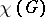of. If,is said to be-chromatic. A graph is-chromatic if and only if it contains no simple cycles of odd length. If the maximum degree of the vertices ofis,is always-colourable except in two cases: 1)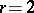andhas a connected component which is a cycle of odd length; or 2)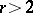and the complete graph withvertices is a connected component of.

The following inequality is valid for the union of two graphsand: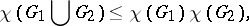equality being attainable. Moreover, ifis such that, then there exist subgraphs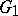and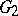insuch that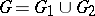,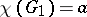,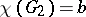. Ifis a graph withvertices and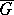is the complementary graph to, then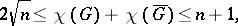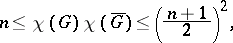all the bounds being attainable. The chromatic numberof a two-dimensional surfaceis the largest of the chromatic numbers of the graphs which can be regularly imbedded in(cf. Graph imbedding). The equality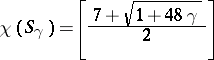is valid for an orientable surfaceof genus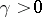. If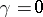, this equation assumes the form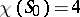, which is the statement of the four-colour problem. Let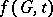be the number of different regular colourings of a graphwith numbered vertices withor fewer colours; then, for any graph, the functionis a polynomial in the variable, called the chromatic polynomial of. Thus, the chromatic polynomial of any tree withvertices has the form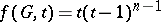. The edge chromatic number (chromatic class)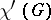ofis the smallest number of colours sufficient for a regular colouring of the edges of. If the maximum degree of the vertices ofis(multiple edges are permitted), then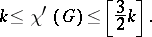If, in addition, the multiplicity of each edge is at most, then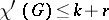. In particular, for graphs without loops or multiple edges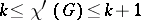.

Problems involving graph colouring arise in design of communications, in radio-electronics, in planning of experiments, and in other fields.

How to Cite This Entry:
Graph colouring. Encyclopedia of Mathematics. URL: http://encyclopediaofmath.org/index.php?title=Graph_colouring&oldid=19036
This article was adapted from an original article by V.B. Alekseev (originator), which appeared in Encyclopedia of Mathematics - ISBN 1402006098. See original article